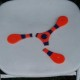Actions

## Feature #8321

open### Ripper: I would like coordinates for keywords

Status:
Open
Priority:
Normal
Assignee:
-
Target version:
-
[ruby-core:54559]

Description

=begin
Ripper gives the (({[line, column]})) coordinates for identifiers, strings, and numbers.

I would like it if it appended those coordinates to most of the block keywords,
including (({:program})), (({:if})), (({:while})), (({:unless})), (({:end})), (({:def})), (({:class})), (({:module})), etc. As with the
identifiers, it should go at the end. So an (({if}))-block would be represented as
 :if
 CONDITION
 BLOCK
 [:elsif, ...] || [:else, ...] || nil
 [lineNo, colNo] # location of the leading :if/:elsif/:else/:unless

I currently get the first coordinate of ((%CONDITION%)), and then look up the preceding
(({:if}))/(({:elsif}))/(({:else})) using (({Ripper.lex(src).find_all{|posn kwd name| kwd == :on_kw && %w/if else elsif/.include?(name) }}))

So the info is in Ripper. It would be more convenient if I could get that info in the src tree.

Note that my suggestion won't break (most) existing code, as the new data goes at the end
of the list.

The same would be useful for other keywords, including (({:module})) (({:class})) (({:def})) (({:try})) (({:catch})) (({:begin})) (({:rescue})).
=end

Actions

Also available in: Atom PDF